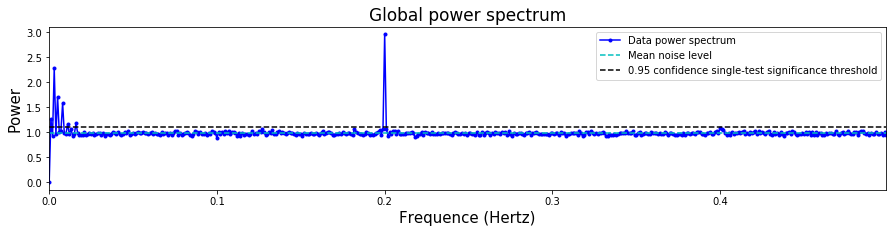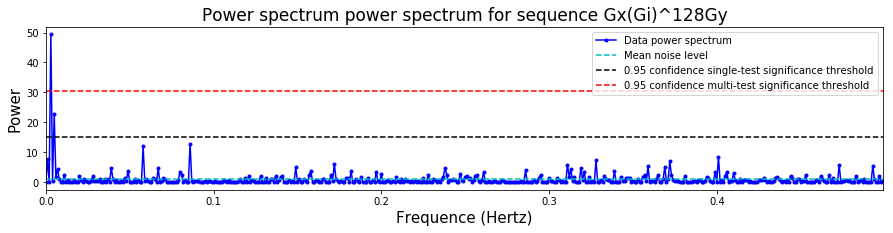# Drift detection and characterization¶

This tutorial shows how to implement drift detection, and a limited characterization, on time-stamped data. This data can be from almost any quantum circuit based experiment on one or more qubits, as long as the data is taken using a suitable time-ordering of the experiments, and the data is recorded as a time series. For example, possible experiments include suitably time-ordered GST, RPE, Ramsey or RB experiments.

This notebook is an introduction to these tools, and it will be either augmented with further notebooks, or updated to be more comprehensive, at a later date.

In :
from __future__ import print_function

# Importing the drift module is essential
from pygsti.extras import drift

# Importing all of pyGSTi is optional, but often useful.
import pygsti


## A quick overview¶

We now give a quick overview of the drift detection and characterization methods in the drift module. Further details are given later in this tutorial. As we demonstrate below, the analysis can be implemented with only two steps:

1. Import some timestamped data, into a pyGSTi dataset.
2. Pass this data to a single analysis function, drift.do_basic_drift_characterization().

Here we demonstrate this with time series GST data, on the $G_i$, $G_x$, $G_y$ gateset, generated from a simulation (the code required to run these simulations is not currently available in pyGSTi). In this simulation the $G_i$ gate has low-frequency drift, the $G_x$ has high-frequency drift, and the $G_y$ gate is drift-free (where "low" and "high" frequency are with respect to the sample rate). More details on the input data format are given later.

In :
ds = pygsti.io.load_tddataset("tutorial_files/timeseries_data.txt")

Loading tutorial_files/timeseries_data.txt: 100%

In :
# This takes 5 - 10 minutes, but can be sped up a lot with more user input (see below)
results_gst = drift.do_basic_drift_characterization(ds)


Thats it!

Everything has been calculated, and we can now look at the results.

Is there any detectable drift?

One useful result is printed below: a yes/no outcome for whether or not drift is detected. This is calculated using multiple statistical tests on the data at a specified global confidence level (which defaults to 0.95 when no user-specified value is passed to drift.do_basic_drift_characterization). That is, here there is a probability of at most 0.05 that this function will report drift when there is none.

In :
results_gst.any_drift_detect()

Statistical tests set at a global confidence level of: 0.95
Result: The 'no drift' hypothesis *is* rejected.


We can plot power spectra

These spectra should be flat - up to statistical flucations, due to finite-sampling noise, around the mean noise level - if there is no drift. There are a range of power spectra that we can plot, but the most useful for an overview of the data is the "global power spectrum", obtained from averaging power spectra calculated from the individual data for each of the different gate sequences (again, details on exactly what this is are given later). This is plotted below. If there are peaks above the significance threshold, this power spectra provides statistically significant evidence of drift.

In :
results_gst.plot_power_spectrum()We can extract the drift frequencies

If we have detected drift, we would probably likely like to know the frequencies of the drift. This information can be extracted from the results object as shown below. All frequencies will be in Hz if the timestamps have been provided in seconds (again, details later). Note that these are the frequencies in the drifting outcome probabilities -- they are not directly the frequencies of drift in, say, a Hamiltonian parameter. However, they are closely related to those frequencies.

In :
print(results_gst.global_drift_frequencies)

[0.001 0.003 0.005 0.008 0.011 0.016 0.2  ]


Is there drift for a particular sequence?

There are individual power spectra for all of the sequences. E.g., if we are interested in whether the $G_xG_i^{128}G_y$ sequence shows signs of drift, we can plot the power spectrum:

In :
# The gatestring we are interested
gstr = pygsti.objects.GateString(None,'Gx(Gi)^128Gy')
# We hand the gatestring to the plotting function
results_gst.plot_power_spectrum(sequence=gstr,loc='upper right')Box-plots for GST data

If the data is from GST experiments, or anything with a GST-like structure of germs and fudicials, we can create a box-plot which shows the maximum power in the spectrum for each sequence. This maximum power is a reasonable proxy for comparing how "drifty" the data from the different sequences appears to be. But note that the maximum power should not be used to directly compare the level of drift in two different datasets with different parameters, particularly if the number of timestamps is different - because this maximum power will increase with more data, for a fixed level of drift. More on this at a later date.

In the plot below we see that the amount of drift appears to be increasing with sequence length, as would be expected with gate drift. Without performing a detailed analysis, by eye it is clear that the $G_i$ gate is the most drifty, that the $G_x$ gate has some drift, and that the data looks consistent with a drift-free $G_y$ gate.

In :
# This box constructs some GST objects, needed to create any sort of boxplot with GST data
from pygsti.construction import std1Q_XYI # The gateset used with the GST data we imported

# This manually specifies the germ and fiducial structure for the imported data.
fiducial_strs = ['{}','Gx','Gy','GxGx','GxGxGx','GyGyGy']
germ_strs = ['Gi','Gx','Gy','GxGy','GxGyGi','GxGiGy','GxGiGi','GyGiGi','GxGxGiGy','GxGyGyGi','GxGxGyGxGyGy']
log2maxL = 9 # log2 of the maximum germ power

# Below we use the maxlength, germ and fuducial lists to create the GST structures needed for box plots.
fiducials = [pygsti.objects.GateString(None,fs) for fs in fiducial_strs]
germs = [pygsti.objects.GateString(None,gs) for gs in germ_strs]
max_lengths = [2**i for i in range(0,log2maxL)]
gssList = pygsti.construction.make_lsgst_structs(std1Q_XYI.gates, fiducials, fiducials, germs, max_lengths)

In :
# Create a workspace to show the boxplot
w = pygsti.report.Workspace()
w.init_notebook_mode(connected=False, autodisplay=True)